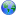Last update January 30, 2008

# Dcalled From CCalling D functions from CExample D functionProblem and SolutionWorking C programOutputPuzzle and /DiscussionAnother Example (under linux)

###Calling D functions from CThis is possible although not emphasised with a heading - see the Interfacing page (D 2.x,D 1.x) in the D language description. All that is needed is to declare the function as`extern (C)`

###Example D function```/* Compute the greatest common divisor of positive integers */ extern(C) { int gcx (int x, int y) { int g; g = y; while (x > 0) { g = x; x = y % x; y = g; } return g; } }```

###Problem and SolutionThe problem is that a C program which calls only this, does not link as there are undefined references _deh_beg and _deh_end . To resolve these it is necessary to define and call a D main program. This can be done in a routine callable from C.```extern(C) int call_main() { return main(); } int main() { printf("Hello from main \n"); return 0; }```

###Working C program```#include int call_main(); int gcx(int x, int y); int main() { printf("hello world\n"); int x = call_main(); printf("int y = gcx(5,50); calls a D function\n"); int y = gcx(5,50); printf("The result is y = %d\n",y); return x; }```

###Output```hello world Hello from main int y = gcx(5,50); calls a D function The result is y = 5```

###Puzzle and /DiscussionThe above also works with only the definition of the D main() routine and without the call. I am not clear as to whether that is just that this example is so simple. Does the D main() do some housekeeping which is needed? Should there be call to something else at exit, or has it been done when main() exited?

There is indeed some housekeeping going on in dmd/src/phobos/internal/dmain2.d
For some sample code seeNG:digitalmars.com.D.gnu/1436

###Another Example (under linux)Dmaindummy.d`int main(){return 0;}`

Dfunc.d```import std.c.stdio; extern (C) void dfunc() { printf("\nMy code is easier to read and write!\n"); }```

Cmain.c```#include extern dfunc(); int main() { dfunc(); printf("\nld likes my main() better than D's main()!\n"); return 0; }```

Put it all together!```#dmd -c Dmaindummy.d Dfunc.d #gcc Cmain.c DmainDummy.o Dfunc.o -o Ctest -lphobos -lpthread -lm #./Ctest My code is easier to read and write! ld likes my main() better than D's main()!```

| | | | | | | | | |

(date of last change: January 30, 2008 22:15 )